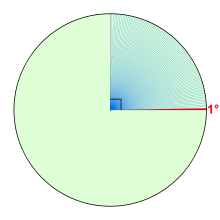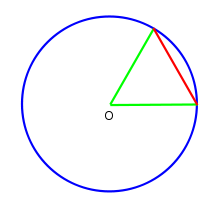# Degree (angle)

Degree (angle)One degree (shown in red) and
ninety degrees (shown in blue)
This article describes the unit of angle. For other meanings and some specific usages, see degree.

A degree (in full, a degree of arc, arc degree, or arcdegree), usually denoted by ° (the degree symbol), is a measurement of plane angle, representing 1360 of a full rotation; one degree is equivalent to π/180 radians. It is not an SI unit, as the SI unit for angles is radian, but it is mentioned in the SI brochure as an accepted unit.

## HistoryA circle with an equilateral chord (red). One sixtieth of this arc is a degree. Six such chords complete the circle.

The original motivation for choosing the degree as a unit of rotations and angles is unknown. One theory states that it is related to the fact that 360 is approximately the number of days in a year. Ancient astronomers noticed that the stars in the sky, which circle the celestial pole every day, seem to advance in that circle by approximately one-360th of a circle, i.e., one degree, each day. Some ancient calendars, such as the Persian calendar, used 360 days for a year. The use of a calendar with 360 days may be related to the use of sexagesimal numbers.

Another theory is that the Babylonians subdivided the circle using the angle of an equilateral triangle as the basic unit and further subdivided the latter into 60 parts following their sexagesimal numeric system. The earliest trigonometry, used by the Babylonian astronomers and their Greek successors, was based on chords of a circle. A chord of length equal to the radius made a natural base quantity. One sixtieth of this, using their standard sexagesimal divisions, was a degree.

Aristarchus of Samos and Hipparchos seem to have been among the first Hellenic scientists to exploit Babylonian astronomical knowledge and techniques systematically. Timocharis, Aristarchus, Aristillus, Archimedes, and Hipparchus were the first Greeks known to divide the circle in 360 degrees of 60 arc minutes (DIO 14 ‡2 p.19 n.24). Eratosthenes used a simpler sexagesimal system dividing a circle into 60 parts.

The division of the circle into 360 parts also occurred in ancient India, as evidenced in the Rigveda:

Twelve spokes, one wheel, navels three.
Who can comprehend this?
On it are placed together
three hundred and sixty like pegs.
They shake not in the least.

Dirghatamas , Rigveda 1.164.48

Another motivation for choosing the number 360 may have been that it is readily divisible: 360 has 24 divisors, including every number from 1 to 10 except 7 . This property has many useful applications, such as dividing the world into 24 time zones, each of which is nominally 15° of longitude, to correlate with the established 24-hour day convention.

Finally, it may be the case that more than one of these factors has come into play. According to that theory, the number is approximately 365 because of the apparent movement of the sun against the celestial sphere and that it was rounded to 360 for some of the mathematical reasons cited above.

## Subdivisions

For many practical purposes, a degree is a small enough angle that whole degrees provide sufficient precision. When this is not the case, as in astronomy or for latitudes and longitudes on the Earth, degree measurements may be written with decimal places like 40.1875° with the degree symbol behind the decimals.

Alternatively, the traditional sexagesimal unit subdivision can be used. One degree is divided into 60 minutes (of arc), and one minute into 60 seconds (of arc). These units, also called the arcminute and arcsecond, are respectively represented as a single and double prime: for example, 40.1875° = 40° 11′ 15″ . Sometimes single and double quotation marks are used instead 40° 11' 15" .

If still more accuracy is required, current practice is to use decimal divisions of the second like 40° 11′ 15.4″ . The older system of thirds, fourths, etc., which continues the sexagesimal unit subdivision, was used by al-Kashi and other ancient astronomers, but is rarely used today. These subdivisions were denoted[citation needed] by writing the Roman numeral for the number of sixtieths in superscript: 1I for a "prime" (minute of arc), 1II for a second, 1III for a third, 1IV for a fourth, etc. Hence the modern symbols for the minute and second of arc, and the word "second" also refer to this system.[citation needed]

## Alternative unitsA chart to convert between degrees and radians

In most mathematical work beyond practical geometry, angles are typically measured in radians rather than degrees. This is for a variety of reasons; for example, the trigonometric functions have simpler and more "natural" properties when their arguments are expressed in radians. These considerations outweigh the convenient divisibility of the number 360. One complete turn (360°) is equal to 2π radians, so 180° is equal to π radians, or equivalently, the degree is a mathematical constant: 1° = π180.

The turn (or revolution, full circle, full rotation, cycle) is used in technology and science. 1 turn = 360°.

With the invention of the metric system, based on powers of ten, there was an attempt to define a "decimal degree" (grad or gon), so that the number of decimal degrees in a right angle would be 100 gon, and there would be 400 gon in a circle. Although this idea was abandoned already by Napoleon, some groups have continued to use it and many scientific calculators still support it.

An angular mil, which is most used in military applications, has at least three specific variants, ranging from 16400 to 16000, each approximately equal to one milliradian. However, 16000 used by the Russian Army originated in Imperial Russia, where an equilateral chord was divided into tenths to give a circle of 600 units (this may be seen on a uglomer,[clarification needed] circa 1900, in the St Petersberg Museum of Artillery).

### Conversion of some common angles

Units Values
Turns   0 112 18 16 14 12 34 1
Degrees   30° 45° 60° 90° 180° 270° 360°
Radians 0$\tfrac{\pi}{6}$$\tfrac{\pi}{4}$$\tfrac{\pi}{3}$$\tfrac{\pi}{2}$ π$\tfrac{3\pi}{2}$
Grads 0g 33⅓g 50g 66⅔g 100g 200g 300g 400g

Wikimedia Foundation. 2010.

### Look at other dictionaries:

• Degree — may refer to: Contents 1 As a unit of measurement 2 In mathematics 3 In education …   Wikipedia

• Degree (mathematics) — This article is about the term degree as used in mathematics. For alternative meanings, see Degree (disambiguation). In mathematics, there are several meanings of degree depending on the subject. Contents 1 Unit of angle 2 Degree of a monomial 3… …   Wikipedia

• Angle trisection — The problem of trisecting the angle is a classic problem of compass and straightedge constructions of ancient Greek mathematics.Two tools are allowed # An un marked straightedge, and # a compass, Problem: construct an angle one third a given… …   Wikipedia

• angle — 01. Japanese people bow at different [angles] to show different meaning. 02. A horse has to change the [angle] of its head to focus it eye instead of changing the shape of the lens of the eye as we do. 03. The floor of the old house is [angled] a …   Grammatical examples in English

• angle — [[t]æ̱ŋg(ə)l[/t]] ♦♦♦ angles, angling, angled 1) N COUNT An angle is the difference in direction between two lines or surfaces. Angles are measured in degrees. → See also right angle The boat is now leaning at a 30 degree angle. 2) N COUNT: usu… …   English dictionary

• angle — an|gle1 [ æŋgl ] verb transitive 1. ) to make something point in a particular direction, especially one that is not directly in front of you: Angle the mirror so that you can see behind you. a ) to make a ball move in a direction that is not… …   Usage of the words and phrases in modern English

• degree*/*/*/ — [dɪˈgriː] noun 1) [C] a unit for measuring temperature, that is often shown as a number followed by the symbol ° It will probably be a few degrees colder by the weekend.[/ex] 2) [C] a unit for measuring angles, that is often shown as a number… …   Dictionary for writing and speaking English

• Degree of curvature — This article is about the measure of curvature. For other uses, see degree (angle). Degree of curve or degree of curvature is a measure of curvature of a circular arc used in civil engineering for its easy use in layout surveying. A n degree… …   Wikipedia

• degree — [di grē′] n. [ME degre < OFr degré, degree, step, rank < VL * degradus < degradare: see DEGRADE] 1. any of the successive steps or stages in a process or series 2. a step in the direct line of descent [a cousin in the second degree] 3.… …   English World dictionary

• Degree — De*gree , n. [F. degr[ e], OF. degret, fr. LL. degradare. See {Degrade}.] 1. A step, stair, or staircase. [Obs.] [1913 Webster] By ladders, or else by degree. Rom. of R. [1913 Webster] 2. One of a series of progressive steps upward or downward,… …   The Collaborative International Dictionary of English